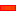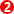﻿ Dialog: CAD-Line | Mass Properties tab

# Dialog: CAD-Line | Mass Properties tab

## CAD-Line | Mass Properties tab

See also : CAD-Line dialog-box

MechDesigner has three sources of Mass-Properties:

 • Mass-Properties that are user-defined on the CAD-Line.
 • Mass Properties imported with a SolidWorks document.
 • Mass Properties that are derived from Profile/Extrusions on a Part.

### CAD-Line dialog-box

#### Mass Properties tabMass : (kg) Centre-of-Mass: X–axis (mm) The X-axis is along the CAD-Line, with (0mm) at the start-Point of the Part. Centre-of-Mass: Y–axis (mm) The Y-axis is at +90º from the CAD-Line, with (0mm) at the start-Point of the Part. Inertia If you define the mass on its position, then the mass will be a Point-Mass. Its mass distribution is defined its Radius-of-Gyration (mm) or Moment-of-Inertia (kg.m2) Note: Units: kilogram.metres2 NOT kilogram.millimeter2

If you enter Moment-of-Inertia, then with the Mass, MechDesigner calculates the equivalent Radius-of-GyrationIf you enter Radius-of-Gyration, then with the Mass, MechDesigner calculates the equivalent Moment-of-InertiaParallel Axis Theorem

MechDesigner uses the Parallel Axis Theorem to calculate the Moment-of-Inertia, Io , about the Part's rotational axis:

I0 = IG +M.h2 where h is the distance from the Centre-of-Mass to the rotational axisWhen you import a SolidWorks document onto a CAD-Line, you can also import the SolidWorks Mass-PropertiesButtonIMPORT / UPDATE Mass Properties FROM THE ACTIVE SolidWorks DOCUMENT

Note: Before you click this button, make sure that the  active SolidWorks document is the model on the CAD-Line.

 Mass : (kg) The Mass of the active SolidWorks document SolidWorks Centre-of-Mass : X-axis: (mm) The distance along the X-axis from the CAD-Line start-Point to the Centre-of-Mass. Centre-of-Mass Y-axis (mm) The distance along the Y-axis, from the CAD-Line start-Point to the Centre-of-Mass. Moment-of-Inertia (C.o.G) (kg.m2) The Inertia with respect to the Centre-of-Mass

ButtonRemove SolidWorks Mass PropertiesTotal Mass Properties:

The Total Mass Properties for ALL CAD-Lines in one Part.

EXPLICITLY: the Total Mass Properties include, for each Part, ALL of those:

 • Mass and Mass-Properties as calculated for ALL Profile/Extrusions in the Part
 • User Mass-Properties as defined for ALL CAD-Lines in the Part
 • SolidWorks Mass-Properties as defined for ALL CAD-Lines in the PartNOTES:

Parallel-Axis Theorem

Inertia about Part's Rotational-Axis = Total Inertia about Centre-of-Mass + Mass × (Distance to Part's Rotational-Axis)2

Relationship between Moment-of-Inertia and Radius of Gyration

Total Inertia (about Center-of-Mass) = Total Mass × (Radius-of-Gyration)2

Total Mass of Part =

Mass of all Extrusions + Total mass of other CAD-Lines in the same Part

Total Inertia about the Part's Centre-of-Gravity =

Inertia of all Extrusions + Total Inertia of other CAD-Lines in the same Part

Tutorials and Reference Help Files for MechDesigner and MotionDesigner 14.2 + © Machine, Cam, Mechanism, and Motion Design Software by PSMotion Ltd